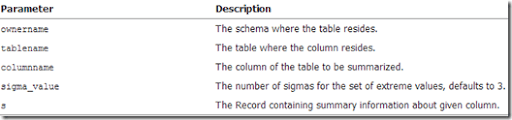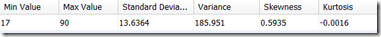### Part 1–Getting started with Statistics for Oracle Data Science projects

Posted on

With all analytics or data science projects one of the first steps typically involves the extraction of data from various sources, merging the data and then performing various statistics.

The extraction and merging of the data is well covered by lots of other people blogging about how to use Oracle Data Integration (ODI), Oracle Warehouse Builder (OWB), among many others.

What I’m going to look at in this series of blog posts will be what statistical functions you might look at using in the Oracle and how to use them.

• This the first blog post in the series will look at the DBMS_STAT_FUNCS PL/SQL package, what it can be used for and I give some sample code on how to use it in your data science projects. I also give some sample code that I typically run to gather some additional stats.
• The second blog post will look at some of the other statistical functions that exist in SQL that you will/may use regularly in your data science projects.
• The third blog post will provide a summary of the other statistical functions that exist in the database.

These statistical functions can be grouped into 2 main types. The first is the descriptive statistics that are available by using the DBMS_STAT_FUNCS PL/SQL package and then there is the extensive list of other SQL stats functions.  It is worth mentioning at this point that all these stats packages and functions come as standard in the database (i.e. they are FREE, you do not have to pay for an add on option for the database to use them). So once you have you Oracle database installed you can start using them. There is no need to spend money buying another stats package to do stats. All you need to know is some SQL and what the stats functions are.

DBMS_STAT_FUNCS

One of the stats package that I use a lot is the SUMMARY function. This is part of the DBMS_STAT_FUNC PL/SQL package. This package calculates a number of common statistics for an attribute in a table. Yes that’s correct, it only gather statistics for just one attribute. So you will have to run it for all the numeric attributes in the table.

For does people who are familiar with the Oracle Data Miner tool, the explore data node produces a lot of these statistics that the SUMMARY function produces. See below for details of how to produce the Histograms.

The SUMMARY function has the following parametersAlthough you will probably be running this this function on the data in your schema you still have to give the schema name. The table name is the name of the table where the data exists, the column name is the name of the column that contains the actual data you want to analyse, and the ‘s’ is the record that will be returned by the SUMMARY function that contains all the summary information.

An example of the basic script to run the SUMMARY function is given below. It will use the data that is available in the sample schemas and the views that where setup for the Oracle Data Mining sample schemas. The table (or in this case the view) that we are going to use is the MINING_DATA_BUILD_V. What we are going to do is to replicate some of what the Explore Node does in the Oracle Data Miner tool.

set serveroutput on

declare
s         DBMS_STAT_FUNCS.SummaryType;
begin

DBMS_STAT_FUNCS.SUMMARY(‘DMUSER’, ‘MINING_DATA_BUILD_V’, ‘AGE’, 3, s);

dbms_output.put_line(‘SUMMARY STATISTICS’);
dbms_output.put_line(‘Count  : ‘||s.count);
dbms_output.put_line(‘Min    : ‘||s.min);
dbms_output.put_line(‘Max    : ‘||s.max);
dbms_output.put_line(‘Range  : ‘||s.range);
dbms_output.put_line(‘Mean   : ‘||round(s.mean));
dbms_output.put_line(‘Mode Count : ‘||s.cmode.count);
dbms_output.put_line(‘Mode        : ‘||s.cmode(1));
dbms_output.put_line(‘Variance    : ‘||round(s.variance));
dbms_output.put_line(‘Stddev      : ‘||round(s.stddev));
dbms_output.put_line(‘Quantile 5  : ‘||s.quantile_5);
dbms_output.put_line(‘Quantile 25 : ‘||s.quantile_25);
dbms_output.put_line(‘Median      : ‘||s.median);
dbms_output.put_line(‘Quantile 75 : ‘||s.quantile_75);
dbms_output.put_line(‘Quantile 95 : ‘||s.quantile_95);
dbms_output.put_line(‘Extreme Count : ‘||s.extreme_values.count);
dbms_output.put_line(‘Extremes      : ‘||s.extreme_values(1));
dbms_output.put_line(‘Top 5 : ‘||s.top_5_values(1)||’,’||
s.top_5_values(2)||’,’||
s.top_5_values(3)||’,’||
s.top_5_values(4)||’,’||
s.top_5_values(5));
dbms_output.put_line(‘Bottom 5 : ‘||s.bottom_5_values(5)||’,’||
s.bottom_5_values(4)||’,’||
s.bottom_5_values(3)||’,’||
s.bottom_5_values(2)||’,’||
s.bottom_5_values(1));
end;
/We can compare this to what is produced by the Explore Node in ODMWe can see that the Explore Node gives us more statistics to help us with understanding the data.

What Statistics does the Explore Node produce

We can see the actual SQL code that the Explore Node runs to get the statistics that are displayed in the Explore Node View Data window. To do this you will need to right-click on the Explore Node and move the mouse down to the Deploy option. The submenu will open and select ‘SQL to Clipboard’ from the list. Open a text editor and past the code. You  will need to tidy up some of this code to point it at the actual data source you want. You will get the following

SELECT /*+ inline */  ATTR,
DATA_TYPE,
NULL_PERCENT,
DISTINCT_CNT,
DISTINCT_PERCENT,
MODE_VALUE,
AVG,
MIN,
MAX,
STD,
VAR,
SKEWNESS,
KURTOSIS,
HISTOGRAMS
FROM OUTPUT_1_23;

Where OUTPUT_1_23 is a working table that ODM has created to store intermediate results from some of its processing. In this case the Explore Node. You will need to change this to the ODM working table in your schema.

This query does not perform any of the statistics gathering. It just presents the results.

Creating our own Statistics gathering script – Part 1

The attribute names in the above SQL query tells us what statistics functions within Oracle that were used. We can replicate this statistics gathering task using the following script. There are four parts to this script. The first part gathers most of the common statistics for the attribute. The second and third parts calculate the Skewness and Kurtosis for the attribute. The final (fourth) part combines the first three parts and lists the outputs.

The one statistic function that we are not including at this point is the Histogram information. I will cover this in the next (second) blog post on statistics.

The following script has the data source table/view name included (MINING_DATA_BUILD_V) and the attribute we are going to use (AGE).  You will need to modify this script to run it for each attribute.

WITH
basic_statistics AS (select (sum(CASE WHEN age IS NULL THEN 1 ELSE 0 END)/COUNT(*))*100 null_percent,
count(*)    num_value,
count(distinct age)   distinct_count,
(count(distinct age)/count(*))*100     distinct_percent,
avg(age)      avg_value,
min(age)      min_value,
max(age)     max_value,
stddev(age)  std_value,
stats_mode(age)   mode_value,
variance(age)       var_value
from   mining_data_build_v),
skewness AS (select avg(SV) S_value
from (select power((age – avg(age) over ())/stddev(age) over (), 3) SV
from mining_data_build_v) ),
kurtosis AS (select avg(KV) K_value
from (select power((age – avg(age) over ())/stddev(age) over (), 4) KV
from mining_data_build_v) )
SELECT null_percent,
num_value,
distinct_percent,
avg_value,
min_value,
max_value,
std_value,
mode_value,
var_value,
S_value,
K_value
from basic_statistics,
skewness,
kurtosis;

Part 2 – Lets do it for all the attributes in a table

In the code above I’ve shown how you can gather the statistics for one particular attribute of one table.But in with an analytics project you will want to gather the statistics on all the attributes.

What we can do is to take the code above and put it into a procedure. This procedure accepts a table name as input, loops through the attributes for this table and calculates the various statistics. The statistics are saved in a table called DATA_STATS (see below).

drop table data_stats;

create table DATA_STATS (
table_name VARCHAR2(30) NOT NULL,
column_name VARCHAR2(30) NOT NULL,
data_type VARCHAR2(106) NOT NULL,
data_length NUMBER,
data_percision NUMBER,
data_scale NUMBER,
num_records NUMBER,
distinct_count NUMBER,
null_percent NUMBER,
distinct_percent NUMBER,
avg_value NUMBER,
min_value NUMBER,
max_value NUMBER,
std_value NUMBER,
mode_value VARCHAR2(1000),
var_value NUMBER,
s_value NUMBER,
k_value NUMBER,
PRIMARY KEY (table_name, column_name));

This is one of the first things that I do when I start on a new project. I create the DATA_STATS table and run my procedure GATHER_DATA_STATS for each table that we will be using. By doing this it allows me to have a permanent records of the stats for each attribute and saves me time in having to rerun various stats at different points of the project. I can also use these stats to produces some additional stats or to produce some graphs.

He is the code for the GATHER_DATA_STATS procedure.

CREATE OR REPLACE PROCEDURE gather_data_stats(p_table_name IN varchar2) AS

cursor c_attributes (c_table_name varchar2)
is SELECT table_name,
column_name,
data_type,
data_length,
data_precision,
data_scale
FROM user_tab_columns
WHERE table_name = upper(c_table_name);

v_sql     NUMBER;
v_rows    NUMBER;
BEGIN
dbms_output.put_line(‘Starting to gather statistics for ‘||upper(p_table_name)||’ at ‘||to_char(sysdate,’DD-MON-YY HH24:MI:SS’));

FOR r_att in c_attributes(p_table_name) LOOP
—
— remove any previously generated stats
—
v_sql := DBMS_SQL.OPEN_CURSOR;
DBMS_SQL.PARSE(v_sql, ‘delete from DATA_STATS where table_name = ”’||r_att.table_name||”’ and column_name = ”’||r_att.column_name||””, DBMS_SQL.NATIVE);
v_rows := DBMS_SQL.EXECUTE(v_sql);
–dbms_output.put_line(‘delete from DATA_STATS where table_name = ”’||r_att.table_name||”’ and column_name = ”’||r_att.column_name||””);

IF r_att.data_type = ‘NUMBER’ THEN
dbms_output.put_line(r_att.table_name||’ : ‘||r_att.column_name||’ : ‘||r_att.data_type);

—
— setup the insert statement and execute
—
v_sql := DBMS_SQL.OPEN_CURSOR;
DBMS_SQL.PARSE(v_sql, ‘insert into data_stats select ”’||r_att.table_name||”’, ”’||r_att.column_name||”’, ”’||r_att.data_type||”’, ‘||r_att.data_length||’, ‘||nvl(r_att.data_precision,0)||’, ‘||nvl(r_att.data_scale,0)||’, count(*) num_value, (sum(CASE WHEN ‘||r_att.column_name||’ IS NULL THEN 1 ELSE 0 END)/COUNT(*))*100 null_percent, count(distinct ‘||r_att.column_name||’) distinct_count, (count(distinct ‘||r_att.column_name||’)/count(*))*100 distinct_percent, avg(‘||r_att.column_name||’) avg_value, min(‘||r_att.column_name||’) min_value, max(‘||r_att.column_name||’) max_value, stddev(‘||r_att.column_name||’) std_value, stats_mode(‘||r_att.column_name||’) mode_value, variance(‘||r_att.column_name||’) var_value, null, null from ‘|| r_att.table_name, DBMS_SQL.NATIVE);
v_rows := DBMS_SQL.EXECUTE(v_sql);

ELSIF r_att.data_type IN (‘CHAR’, ‘VARCHAR’, ‘VARCHAR2’) THEN
dbms_output.put_line(r_att.table_name||’ : ‘||r_att.column_name||’ : ‘||r_att.data_type);

—
— We need to gather a smaller number of stats for the character attributes
—
v_sql := DBMS_SQL.OPEN_CURSOR;
begin

DBMS_SQL.PARSE(v_sql, ‘insert into data_stats select ”’||r_att.table_name||”’, ”’||r_att.column_name||”’, ”’||r_att.data_type||”’, ‘||r_att.data_length||’, ‘||nvl(r_att.data_precision,0)||’, ‘||nvl(r_att.data_scale,0)||’, count(*) num_value, (sum(CASE WHEN ‘||r_att.column_name||’ IS NULL THEN 1 ELSE 0 END)/COUNT(*))*100 null_percent, count(distinct ‘||r_att.column_name||’) distinct_count, (count(distinct ‘||r_att.column_name||’)/count(*))*100 distinct_percent, null, null, null, null, stats_mode(‘||r_att.column_name||’) mode_value, null, null, null from ‘|| r_att.table_name, DBMS_SQL.NATIVE);
v_rows := DBMS_SQL.EXECUTE(v_sql);

— dbms_output.put_line(‘insert into data_stats select ”’||r_att.table_name||”’, ”’||r_att.column_name||”’, ”’||r_att.data_type||”’, ‘||r_att.data_length||’, ‘||nvl(r_att.data_precision,0)||’, ‘||nvl(r_att.data_scale,0)||’, count(*) num_value, (sum(CASE WHEN ‘||r_att.column_name||’ IS NULL THEN 1 ELSE 0 END)/COUNT(*))*100 null_percent, count(distinct ‘||r_att.column_name||’) distinct_count, (count(distinct ‘||r_att.column_name||’)/count(*))*100 distinct_percent, null, null, null, null, stats_mode(‘||r_att.column_name||’) mode_value, null, null, null from ‘|| r_att.table_name);
exception
when others then
dbms_output.put_line(v_rows);
end;

ELSE
dbms_output.put_line(‘Unable to gather statistics for ‘||r_att.column_name||’ with data type of ‘||r_att.data_type||’.’);
END IF;
END LOOP;

dbms_output.put_line(‘Finished gathering statistics for ‘||upper(p_table_name)||’ at ‘||to_char(sysdate,’DD-MON-YY HH24:MI:SS’));

commit;
END;

Then to run it for a table:

exec gather_data_stats(‘mining_data_build_v’);

We can view the contents of the DATA_STATS table by executing the following in SQL*Plus or SQL Developer

select * from DATA_STATS;